Home Page Introduction Classical Ciphers Enigma Machine DES Key Agreement PGP R.S.A. Number Theory Other Links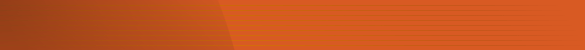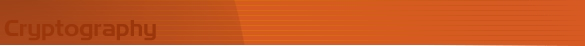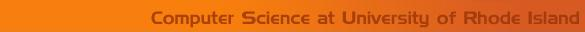Classical Cryptography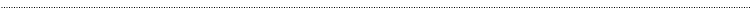Vigenere Cipher Cryptanalysis

The Vigenere Cipher initially seems very secure, however it can be broken fairly easily once the length of the keyword is known. If you know that the length of the keyword is n, you can break the ciphertext into n cosets and attack the cipher using frequency analysis if the ciphertext sample is long enough. This page will look at two methods to determine the length of the keyword, the Friedman and Kasiski tests.

Friedman Test

 Finding the Incidence of Coincidence for a sample of ciphertext can indicate whether or not a polyaphabetic substitution has been used to encipher a message. The Incidence of Coincidence is the probablility that two randomly selected letters are the same. Ordinary English has an incidence of coincidence of 0.065. If a sample of text has an incidence of coincidence close to this number it is likely to be a monoalphabetic substitution, if it is larger then 0.0385 and less then 0.065 the text was most likely enciphered using a polyalphabetic cipher like the Vigenere cipher. The formula for finding the incidence of coincidence is: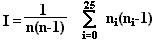where n0, n1, n2, ..., n24, n25 are the respective counts of letters A,B,C,...,Y,Z in the sample of ciphertext and n=n0 + n1 + n2 + ... + n24 + n25 (the total number of letters in the ciphertext). To find the estimated keyword length, calculate the value of: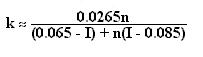Kasisiki Text

The Kasiski Test uses the occasional aligning of groups of letters with the keyword to determine the length of the keyword. This will produce repeated groups of letters in the ciphertext. By counting the number of letters between the beginnings of these repeated groups of letters and finding a number which is the multiple of those distances, we can estimate the length of the keyword.
Example:

 plain: THECHILDISTHEFATHEROFTHEMAN key: POETRYPOETRYPOETRYPOETRY ciphertext: IVEVYGARMLMYIVEKFDIVEFRL

The second group of repeated letters IVE occurs 12 letters after the first. The third group of letters appears 6 letters after the first. Since both of these numbers are multiples of 6, this suggests that the length of the keyword is 6.